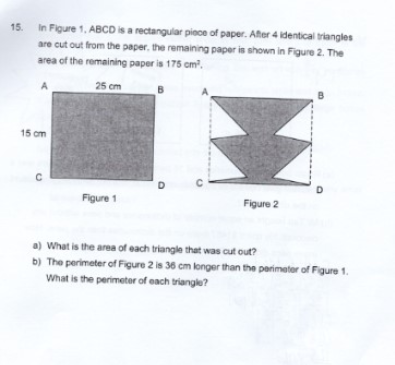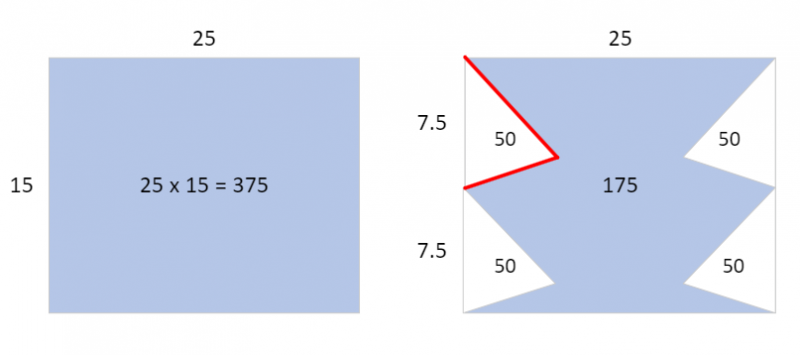# QuestionHow to tackle this question?

a)

Area of rectangle ABCD = 25*15= 375 cm square

4* Area of Cut triangle = 375-175=200

Area of Cut triangle = 50 cm square

b)

The perimeter of figure 2 is 36 cm more than figure 1 .

Since AB and CD are the length same in both perimeter, we can ignore the length.

The perimeter of figure2 AC and BD added up to  be 15*2+36=66.

66/4 = 16.5 is the length of 2 sides of the cut triangle.

So the perimeter of the cut triangle is 16.5+15/2=24cm.

0 Replies 1 Like```Part (a)
Area of Fig1 = 25 x 15 = 375
Area of Fig2 = 175
Area of 4 triangles = Area of Fig1 - Area of Fig2
= 375 - 175 = 200
Area of 1 triangle  = 200/4 = 50 cm2

Part (b)
Let the red segments be of length u cm. There are 4 of them.
Perimeter of Fig2 = 25 + 25 + 4u      = 50 + 4u
Perimeter of Fig1 = 25 + 25 + 15 + 15 = 50 + 30
Difference = 4u - 30 = 36
So u = (36 + 30)/4 = 16.5
Perimeter of 1 triangle = 7.5 + u = 7.5 + 16.5 = 24 cm
```
0 Replies 1 Like

(a) Area of rect.  paper = 25 x 15 = 375

Area of paper cut out = Area of 4 triangles  = 375 – 175 = 200

Hence Area of each triangle = 200/4 = 50cm2

(b)  Perimeter of rect. paper  = 2(25+15) = 80

Perimeter of figure 2 = 36 + 80 = 116

Perimeter of figure 2 minus the 2 25-sides = 116 – 50 = 66

The 2-slant-sides of the each triangle = 66/4 = 16.5

The base of each triangle = 15/2 = 7.5

Hence the perimeter of each triangle = 16.5+7.5 = 24cm

0 Replies 1 Like